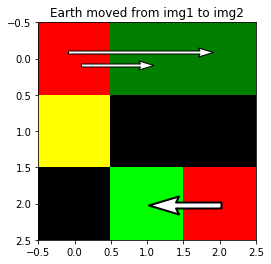# Earth Mover’s Distance in Python

I was exploring the Earth mover’s distance and did some head-scratching on the OpenCV v3  implementation in Python. Here’s some code to hopefully reduce head-scratching for others.  (Fun fact, OpenCV’s Python bindings are automatically generated, so Python documentation isn’t guaranteed. While I found a little bit for the OpenCV 2 implementation, I couldn’t find any for the OpenCV 3 version.)

```import cv2
import numpy as np
import matplotlib.pyplot as plt
```

The EMD function expects “signatures” rather than images/matrices. For an N-dimensional matrix with a total of M elements, the signature is an M x (N+1) array. Each of the M rows corresponds to a single pixel/element in the original image/matrix. Within each row, the first value is the pixel/element value that occurs in the source image/matrix, and the remaining N values are the coordinates along each dimension of that pixel/element. In short, it’s a list of pixel values and their corresponding coordinates.

We can generate this signature by iterating through the values in our source image and filling in the signature’s rows one-by-one. The order we go through the source image pixels doesn’t matter.

In :
```def img_to_sig(arr):
"""Convert a 2D array to a signature for cv2.EMD"""

# cv2.EMD requires single-precision, floating-point input
sig = np.empty((arr.size, 3), dtype=np.float32)
count = 0
for i in range(arr.shape):
for j in range(arr.shape):
sig[count] = np.array([arr[i,j], i, j])
count += 1
return sig
```
In :
```arr1 = np.array([[2, 0, 0],
[2, 0, 0],
[0, 0, 2]])

arr2 = np.array([[0, 1, 1],
[2, 0, 0],
[0, 2, 0]])

sig1 = img_to_sig(arr1)
sig2 = img_to_sig(arr2)

print(sig1)
print(sig2)
```
```[[2. 0. 0.]
[0. 0. 1.]
[0. 0. 2.]
[2. 1. 0.]
[0. 1. 1.]
[0. 1. 2.]
[0. 2. 0.]
[0. 2. 1.]
[2. 2. 2.]]
[[0. 0. 0.]
[1. 0. 1.]
[1. 0. 2.]
[2. 1. 0.]
[0. 1. 1.]
[0. 1. 2.]
[0. 2. 0.]
[2. 2. 1.]
[0. 2. 2.]]
```

The output of `cv2.EMD` has three values. The first is the distance between the two signatures. This appears to be normalized in some way—adding non-moving elements will reduce the distance, and doubling all pixel values doesn’t affect the distance. I’m not sure what the second element—a `None`—is. The third value is the “flow matrix”, telling you what was moved where. Per the documentation, if the input arrays (before being converted to signatures) have `size1` and `size2` elements each, the flow is a “`𝚜𝚒𝚣𝚎𝟷×𝚜𝚒𝚣𝚎𝟸` flow matrix: `𝚏𝚕𝚘𝚠_i,j` is a flow from i-th point of signature1 to j-th point of signature2 .”

In :
```dist, _, flow = cv2.EMD(sig1, sig2, cv2.DIST_L2)

print(dist)
print(_)
print(flow)
```
```0.8333333134651184
None
[[0. 1. 1. 0. 0. 0. 0. 0. 0.]
[0. 0. 0. 0. 0. 0. 0. 0. 0.]
[0. 0. 0. 0. 0. 0. 0. 0. 0.]
[0. 0. 0. 2. 0. 0. 0. 0. 0.]
[0. 0. 0. 0. 0. 0. 0. 0. 0.]
[0. 0. 0. 0. 0. 0. 0. 0. 0.]
[0. 0. 0. 0. 0. 0. 0. 0. 0.]
[0. 0. 0. 0. 0. 0. 0. 0. 0.]
[0. 0. 0. 0. 0. 0. 0. 2. 0.]]
```

So that 1 in row 1, column 2 is saying that one unit of “earth” was moved from the coordinates in row 1 of the first signature, or (0, 0), to the coordinates in row 2 of the second signature, or (0, 1). Note that unmoved earth shows up in this flow matrix—along the diagonal in this case, since the coordinates are in the same order in the two signatures.

Now to visualize this.

In :
```def plot_flow(sig1, sig2, flow, arrow_width_scale=3):
"""Plots the flow computed by cv2.EMD

plotted in a combined image, with the first image in the
red channel and the second in the green. Arrows are
overplotted to show moved earth, with arrow thickness
indicating the amount of moved earth."""

img1 = sig_to_img(sig1)
img2 = sig_to_img(sig2)
combined = np.dstack((img1, img2, 0*img2))
# RGB values should be between 0 and 1
combined /= combined.max()
print('Red channel is "before"; green channel is "after"; yellow means "unchanged"')
plt.imshow(combined)

flows = np.transpose(np.nonzero(flow))
for src, dest in flows:
# Skip the pixel value in the first element, grab the
# coordinates. It'll be useful later to transpose x/y.
start = sig1[src, 1:][::-1]
end = sig2[dest, 1:][::-1]
if np.all(start == end):
# Unmoved earth shows up as a "flow" from a pixel
# to that same exact pixel---don't plot mini arrows
# for those pixels
continue

# Add a random shift to arrow positions to reduce overlap.
shift = np.random.random(1) * .3 - .15
start = start + shift
end = end + shift

mag = flow[src, dest] * arrow_width_scale
plt.quiver(*start, *(end - start), angles='xy',
scale_units='xy', scale=1, color='white',
edgecolor='black', linewidth=mag/3,
width=mag, units='dots',

plt.title("Earth moved from img1 to img2")

def sig_to_img(sig):
"""Convert a signature back to a 2D image"""
intsig = sig.astype(int)
img = np.empty((intsig[:, 1].max()+1, intsig[:, 2].max()+1), dtype=float)
for i in range(sig.shape):
img[intsig[i, 1], intsig[i, 2]] = sig[i, 0]
return img
```
In :
```fig, (ax1, ax2) = plt.subplots(1, 2)
ax1.imshow(arr1, cmap='gray')
ax1.set_title("Starting Distribution")
ax2.imshow(arr2, cmap='gray')
ax2.set_title("Ending Distribution")
plt.show()

plot_flow(sig1, sig2, flow)
```
```Red channel is "before"; green channel is "after"; yellow means "unchanged"```

So we see for these two distributions, 2 units of earth are distributed from the upper-left corner to the other top-row cells, another two units move along the bottom row, and a final two units in the middle row are unmoved.

## One thought on “Earth Mover’s Distance in Python”

1.Fantasys says:

Earth Mover’s Distance can be formulated and solved as a transportation problem. Suppose that several suppliers, each with a given amount of goods, are required to supply several consumers, each with a given limited capacity. For each supplier-consumer pair, the cost of transporting a single unit of goods is given. The transportation problem is then to find a least-expensive flow of goods from the suppliers to the consumers that satisfies the consumers’ demand. Similarly, here the problem is transforming one signature(